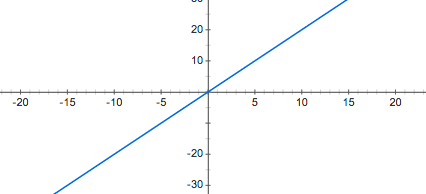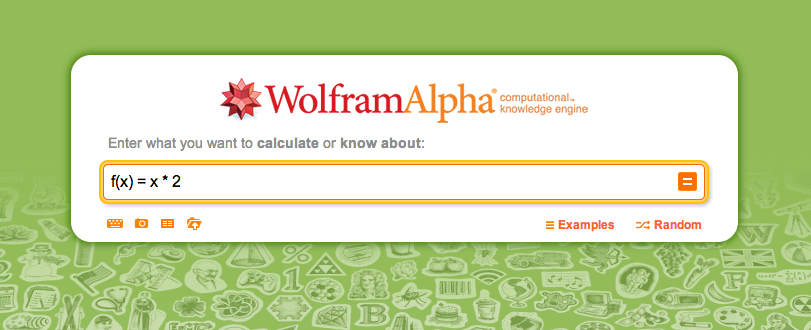# Introduction

As computer programming is based on Mathematics, it is useful to briefly review the idea of mathematical functions, how they are defined, and how they work, before we consider building functions in code. As you will see, the two are closely related and analogous to each other.

# What is a function?

Wikipedia defines a function like this:

"In mathematics, a function is a relation between a set of inputs and a set of permissible outputs with the property that each input is related to exactly one output." (http://en.wikipedia.org/wiki/Function_(mathematics))

A mathematical function has three basic parts:

1. Inputs
2. Process of manipulating the Inputs
3. Outputs

This can be conceptualized as a "function machine":Functions are also defined by an identifier or a label. For example, in the picture above, the "function machine" is labeled as function "f". Considering that the input variable has been named "x", we denote the function in mathematics as f(x), which we say as "'f' of 'x'" (function of the parameter 'x').

An example of the process of manipulating the input would be if we wanted to return the input multiplied by 2. So if the input variable is 'x', we want to return 'x * 2'. We thus use the following mathematical syntax to express a function with this procedure:

``````f(x) = x * 2
``````

Thus, if we substitute x for '4', the function would operate as follows:

``````f(x) = x * 2
let x = 4
f(4) = 4 * 2
f(4) = 8
``````

We can see, that if we input 4 into our "function machine", the output is 8. If we do the same thing with '6', as another example, we see the following:

``````f(x) = x * 2
let x = 6
f(6) = 6 * 2
f(6) = 12
``````

So when the input is 6, the output is 12. If we were to take all numbers between -20 and +20 and put those numbers through our "function machine", we would see an interesting pattern emerge, particularly if we graph the output. Let's take a look at what this would look like.You can try this for yourself using WolframAlpha. On the home page, enter in the function "f(x) = x * 2" and press Enter.WolframAlpha will then give you some information about the function you have entered, as well as graphing the function for you.

# Interpreting the graph of the function

There are some things to notice about the graph above. Firstly, notice that there are three lines in the graph:

1. A black horizontal line
2. A black vertical line
3. A blue diagonal line

The two black lines (1 and 2) are known as the graph's axes. The horizontal line is the x-axis, as this relates to the input variable 'x'. The vertical line is the f(x)-axis as it relates to the output of the function. The blue line, which is really what we are most interested in, shows the relationship between the x and f(x) axis. So, if you use your finger and starting at the '5' marked on the x-axis, trace directly upwards until you hit the blue line, then trace to the left until you hit the f(x) axis, you will see that the corresponding value is '10' on this axis. If we consider that the function is f(x) = x * 2 we can see that this makes perfect sense, as '10' is '5 * 2'.

# Functions as Input

We can also use the output of a function directly as an input for another function. For example, let's say we have the following two functions:

1. f(x) = x^2
2. g(x) = x + 1

Note: "^2" means "raised to the power of 2". Or, in this case, x squared.

Now, if we wanted to pass a value into x, and use the output of f(x) as the input for function g, we could express that mathematically in the following way:

``````g(f(x)) = x + 1, where f(x) = x^2
``````

This might be a bit hard to visualize at first, so let us use our "Function Machine" analogy. To illustrate this, consider the image below:Here, we can see that the value '3' enters the function "f", and is squared, resulting in the output '9', which is then used as the input for function "g", which then has '1' added to it, finally resulting in an output of '10', which is then the result of g(f(x)).

# Exercises

## PART A

Using WolframAlpha, graph the following equations and note the general characterists:

1. f(x) = x^2
2. f(x) = x^3
3. f(x) = -2x^2
4. f(x) = x
5. f(x) = 1/x
6. f(x) = 1/x^2

## PART B

1. Give a mathematical function which is a straight line parallel to the x-axis, and intersects the y-axis at the number 3.
2. Give a mathematical function which is a straight line parallel to the y-axis, and intersects the x-axis at the number 5.
3. Give a mathematical function which takes in a parameter (think variable) called 'z', then manipulates the value of 'z' by multiplying it by 3 and adding 7.
4. (Self research) Define a continuous function.
5. (Self research) What is discrete data?
6. In Python, give an example of a data type which can be used to hold discrete data, and an example of a data type which can be used to hold continuous data.

Continue to next section